Geometrize  1.0 C++ library for geometrizing images into geometric primitives
geometrize::exporter Namespace Reference

## Classes

struct  SVGExportOptions
The SVGExportOptions struct represents the options that can be set for the SVG export. More...

## Enumerations

enum  RotatedEllipseSVGExportMode { RotatedEllipseSVGExportMode::ELLIPSE_ITEM = 0, RotatedEllipseSVGExportMode::POLYGON = 1 }

## Functions

void writeToStream (std::ostringstream &stream, const std::uint8_t &t)

std::string exportBitmapData (const geometrize::Bitmap &bitmapData)
exportBitmapData Exports the raw image data to a binary dump - just the data as RGBA8888, no zero-padding or anything. More...

std::uint16_t flip16 (const std::uint16_t &v)

std::uint32_t flip32 (const std::uint32_t &v)

bool bigEndian ()

void writeToStream (std::ostringstream &stream, const std::uint32_t &t)

void writeToStream (std::ostringstream &stream, const std::uint16_t &t)

std::string exportBMP (const geometrize::Bitmap &bitmapData)
exportBMP Exports the image data to a RGB888 bitmap image file (BMP). More...

std::string exportShapeArray (const std::vector< geometrize::ShapeResult > &data)
exportShapeArray Exports shape data to a compact array-style format. Data is three lines per shape, each line: shapeEnumTypeId shapeData R,G,B,A More...

std::string exportShapeJson (const std::vector< geometrize::ShapeResult > &data)
exportShapeJson Exports shape data to JSON. More...

std::string getSingleShapeSVGData (const geometrize::rgba &color, const geometrize::Shape &shape, SVGExportOptions options=SVGExportOptions{})
getSvgShapeData Gets the SVG data for a single shape. This is just the <rect>/<path> etc block for the shape itself, not a complete SVG image. More...

std::string exportSingleShapeSVG (const geometrize::rgba &color, const geometrize::Shape &shape, const std::uint32_t width, const std::uint32_t height, SVGExportOptions options=SVGExportOptions{})
exportSVG Exports a single shape as a complete SVG image. More...

std::string exportSVG (const std::vector< geometrize::ShapeResult > &data, const std::uint32_t width, const std::uint32_t height, SVGExportOptions options=SVGExportOptions{})
exportSVG Exports shape data as a complete SVG image. More...

## Variables

static const std::string SVG_STYLE_HOOK = "::svg_style_hook::"
SVG_STYLE_HOOK A hook that an SVG exporter should use to augment shape styling produced by the getSvgShapeData method. More...

## Enumeration Type Documentation

 strong
Enumerator
ELLIPSE_ITEM
POLYGON
27 {
28  ELLIPSE_ITEM = 0, // Export as a translated, rotated and scaled svg <ellipse>. OpenFL's SVG library can't handle this
29  POLYGON = 1 // Export as a <polygon>, OpenFL's SVG library can handle this, but it looks quite ugly
30 };

## Function Documentation

 bool geometrize::exporter::bigEndian ( )
inline
25 {
26  std::uint32_t v{1};
27  return (1 != reinterpret_cast<char*>(&v));
28 }

Here is the caller graph for this function: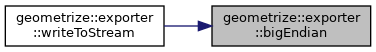std::string geometrize::exporter::exportBitmapData ( const geometrize::Bitmap & bitmapData )

exportBitmapData Exports the raw image data to a binary dump - just the data as RGBA8888, no zero-padding or anything.

Parameters
 bitmapData The image data to save as binary data.
Returns
A string containing the raw bitmap data.
20 {
21  std::ostringstream stream(std::ios::binary);
22
23  for(std::uint32_t y = 0U; y < bitmapData.getHeight(); y++) {
24  for(std::uint32_t x = 0U; x < bitmapData.getWidth(); x++) {
25  const geometrize::rgba pixel(bitmapData.getPixel(x, y));
26  writeToStream(stream, pixel.r);
27  writeToStream(stream, pixel.g);
28  writeToStream(stream, pixel.b);
29  writeToStream(stream, pixel.a);
30  }
31  }
32
33  return stream.str();
34 }
std::uint32_t getWidth() const
getWidth Gets the width of the bitmap.
Definition: bitmap.cpp:20
geometrize::rgba getPixel(std::uint32_t x, std::uint32_t y) const
getPixel Gets a pixel color value.
Definition: bitmap.cpp:40
The rgba struct is a helper for manipulating RGBA8888 color data.
Definition: rgba.h:12
void writeToStream(std::ostringstream &stream, const std::uint8_t &t)
Definition: bitmapdataexporter.cpp:14
std::uint32_t getHeight() const
getHeight Gets the height of the bitmap.
Definition: bitmap.cpp:25

Here is the call graph for this function: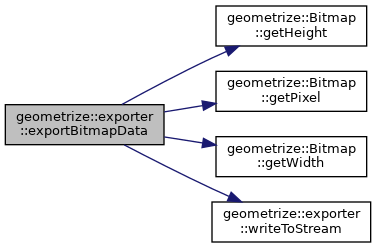std::string geometrize::exporter::exportBMP ( const geometrize::Bitmap & bitmapData )

exportBMP Exports the image data to a RGB888 bitmap image file (BMP).

Parameters
 bitmapData The image data to save as a bitmap image file.
Returns
A string containing the bitmap data.
56 {
57  std::ostringstream stream(std::ios::binary);
58
61
62  const std::uint32_t width{bitmapData.getWidth()}; // The width of the image in pixels.
63  const std::uint32_t height{bitmapData.getHeight()}; // The height of the image in pixels.
64
65  // Per row pad byte count, used to ensure that each row is a multiple of 4 bytes.
66  const std::uint32_t padding{ ((width * 3) % 4 != 0) ? 4 - ((width * 3) % 4) : 0};
67
69  const std::uint32_t ifSize{BITMAP_INFORMATION_HEADER_SIZE}; // The number of bytes required by the structure.
70  const std::uint16_t planes{1U}; // The number of planes for the target device. This value must be set to 1.
71  const std::uint16_t bitCount{24U}; // The number of bits that define each pixel and the maximum number of colors in the bitmap.
72  const std::uint32_t compression{0U}; // Specifies the bitmap compression type.
73  const std::uint32_t imageSize{(((width * 3U) + padding) & 0x0000FFFC) * height}; // The size of the image in bytes.
74  const std::uint32_t xPelsPerMeter{0U}; // The horizontal resolution in pixels-per-meter.
75  const std::uint32_t yPelsPerMeter{0U}; // The vertical resolution in pixels-per-meter.
76  const std::uint32_t colorsUsed{0U}; // The number of color indexes in the color table actually used by the bitmap.
77  const std::uint32_t colorsImportant{0U}; // The number of color indexes required for displaying the bitmap. If zero, all colors are required.
78
80  const std::uint16_t type{19778U}; // The file type.
81  const std::uint32_t fhSize{BITMAP_INFORMATION_HEADER_SIZE + BITMAP_FILE_HEADER_SIZE + imageSize}; // The size in bytes of the bitmap file.
82  const std::uint16_t reserved1{0U}; // Reserved, must be zero.
83  const std::uint16_t reserved2{0U}; // Reserved, must be zero.
84  const std::uint32_t offbits{BITMAP_INFORMATION_HEADER_SIZE + BITMAP_FILE_HEADER_SIZE}; // The offset, in bytes, from the beginning of the BitmapFileHeader structure to the bitmap bits.
85
86  writeToStream(stream, type);
87  writeToStream(stream, fhSize);
88  writeToStream(stream, reserved1);
89  writeToStream(stream, reserved2);
90  writeToStream(stream, offbits);
91
92  writeToStream(stream, ifSize);
93  writeToStream(stream, width);
94  writeToStream(stream, height);
95  writeToStream(stream, planes);
96  writeToStream(stream, bitCount);
97  writeToStream(stream, compression);
98  writeToStream(stream, imageSize);
99  writeToStream(stream, xPelsPerMeter);
100  writeToStream(stream, yPelsPerMeter);
101  writeToStream(stream, colorsUsed);
102  writeToStream(stream, colorsImportant);
103
104  // Bitmap Image Data
105  for(std::uint32_t y = 0U; y < bitmapData.getHeight(); y++) {
106  for(std::uint32_t x = 0U; x < bitmapData.getWidth(); x++) {
107  const geometrize::rgba pixel(bitmapData.getPixel(x, y));
108  writeToStream(stream, pixel.b);
109  writeToStream(stream, pixel.g);
110  writeToStream(stream, pixel.r);
111  }
115  }
116  }
117
118  return stream.str();
119 }
std::uint32_t getWidth() const
getWidth Gets the width of the bitmap.
Definition: bitmap.cpp:20
void writeToStream(std::ostringstream &stream, const std::uint16_t &t)
Definition: bitmapexporter.cpp:40
geometrize::rgba getPixel(std::uint32_t x, std::uint32_t y) const
getPixel Gets a pixel color value.
Definition: bitmap.cpp:40
The rgba struct is a helper for manipulating RGBA8888 color data.
Definition: rgba.h:12
std::uint32_t getHeight() const
getHeight Gets the height of the bitmap.
Definition: bitmap.cpp:25

Here is the call graph for this function: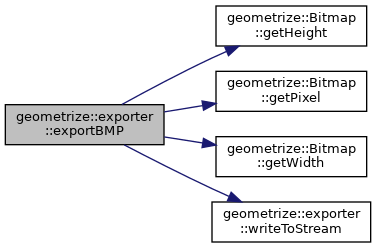std::string geometrize::exporter::exportShapeArray ( const std::vector< geometrize::ShapeResult > & data )

exportShapeArray Exports shape data to a compact array-style format. Data is three lines per shape, each line: shapeEnumTypeId shapeData R,G,B,A

Parameters
 data The shape data to export.
Returns
A string containing the exported data.
19 {
20  std::ostringstream stream;
21
22  for(std::size_t i = 0; i < data.size(); i++) {
23  const geometrize::ShapeResult& s(data[i]);
24
25  stream << static_cast<std::underlying_type<geometrize::ShapeTypes>::type>(s.shape->getType()) << "\n";
26
27  const std::vector<float> shapeData{getRawShapeData(*s.shape.get())};
28  for(std::size_t d = 0; d < shapeData.size(); d++) {
29  stream << shapeData[d];
30  if(d != (shapeData.size() - 1U)) {
31  stream << ",";
32  }
33  }
34  stream << "\n";
35
36  stream << static_cast<std::uint32_t>(s.color.r) << ","
37  << static_cast<std::uint32_t>(s.color.g) << ","
38  << static_cast<std::uint32_t>(s.color.b) << ","
39  << static_cast<std::uint32_t>(s.color.a);
40
41  if(i != (data.size() - 1U)) {
42  stream << "\n";
43  }
44  }
45
46  return stream.str();
47 }
The ShapeResult struct is a container for info about a shape added to the model.
Definition: shaperesult.h:19
std::vector< float > getRawShapeData(const geometrize::Shape &s)
Definition: shapeserializer.cpp:21

Here is the call graph for this function: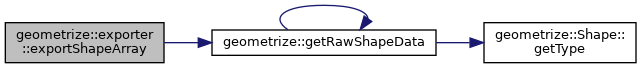std::string geometrize::exporter::exportShapeJson ( const std::vector< geometrize::ShapeResult > & data )

exportShapeJson Exports shape data to JSON.

Parameters
 data The shape data to export.
Returns
A string containing the exported JSON.
20 {
21  std::ostringstream stream;
22  stream << "{\"shapes\":\n[";
23
24  for(std::size_t i = 0; i < data.size(); i++) {
25  const geometrize::ShapeResult& s(data[i]);
26  const geometrize::ShapeTypes type{s.shape->getType()};
27  const std::vector<float> shapeData{getRawShapeData(*s.shape.get())};
28  const geometrize::rgba color(s.color);
29  const double score{s.score};
30
31  stream << "{" << "\"type\":" << static_cast<std::underlying_type<geometrize::ShapeTypes>::type>(type) << ", \"data\":[";
32  for(std::size_t d = 0; d < shapeData.size(); d++) {
33  stream << shapeData[d];
34  if(d <= shapeData.size() - 2) {
35  stream << ",";
36  }
37  }
38  stream << "],\"color\":[" << static_cast<std::uint32_t>(color.r) << "," << static_cast<std::uint32_t>(color.g) << "," << static_cast<std::uint32_t>(color.b) << "," << static_cast<std::uint32_t>(color.a) << "],";
39  stream << "\"score\":" << score << "}";
40
41  if(i <= data.size() - 2) {
42  stream << ",\n";
43  }
44  }
45
46  stream << "\n]}";
47  return stream.str();
48 }
ShapeTypes
The ShapeTypes enum specifies the types of shapes that can be used. These can be combined to produce ...
Definition: shapetypes.h:16
The ShapeResult struct is a container for info about a shape added to the model.
Definition: shaperesult.h:19
std::vector< float > getRawShapeData(const geometrize::Shape &s)
Definition: shapeserializer.cpp:21
The rgba struct is a helper for manipulating RGBA8888 color data.
Definition: rgba.h:12

Here is the call graph for this function: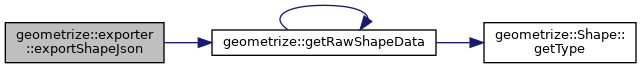std::string geometrize::exporter::exportSingleShapeSVG ( const geometrize::rgba & color, const geometrize::Shape & shape, const std::uint32_t width, const std::uint32_t height, SVGExportOptions options = `SVGExportOptions{}` )

exportSVG Exports a single shape as a complete SVG image.

Parameters
 color The color of the shape to export. shape The shape to export. width The width of the SVG image. height The height of the SVG image. options additional options used by the exporter.
Returns
A string representing the SVG image.
246 {
247  std::stringstream stream;
248
249  stream << "<?xml version=\"1.0\" standalone=\"no\"?>" << "\n";
250  stream << "<svg xmlns=\"http://www.w3.org/2000/svg\" version=\"1.2\" baseProfile=\"tiny\" " <<
251  "width=\"" << width << "\" " << "height=\"" << height << "\" " <<
252  "viewBox=\"" << 0 << " " << 0 << " " << width << " " << height << "\">" << "\n";
253
254  stream << ::getSingleShapeSVGData(color, shape, options);
255
256  stream << "</svg>";
257
258  return stream.str();
259 }
std::string getSingleShapeSVGData(const geometrize::rgba &color, const geometrize::Shape &shape, SVGExportOptions options)
getSvgShapeData Gets the SVG data for a single shape. This is just the <rect>/<path> etc block for th...
Definition: svgexporter.cpp:240

Here is the call graph for this function: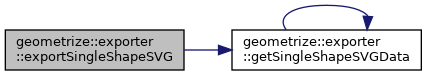std::string geometrize::exporter::exportSVG ( const std::vector< geometrize::ShapeResult > & data, const std::uint32_t width, const std::uint32_t height, SVGExportOptions options = `SVGExportOptions{}` )

exportSVG Exports shape data as a complete SVG image.

Parameters
 data The shape data to export. width The width of the SVG image. height The height of the SVG image. options additional options used by the exporter.
Returns
A string representing the SVG image.
262 {
263  std::stringstream stream;
264
265  stream << "<?xml version=\"1.0\" standalone=\"no\"?>" << "\n";
266  stream << "<svg xmlns=\"http://www.w3.org/2000/svg\" version=\"1.2\" baseProfile=\"tiny\" " <<
267  "width=\"" << width << "\" " << "height=\"" << height << "\" " <<
268  "viewBox=\"" << 0 << " " << 0 << " " << width << " " << height << "\">" << "\n";
269
270  for(std::size_t i = 0; i < data.size(); i++) {
271  options.itemId = i;
272
273  const geometrize::ShapeResult& s(data[i]);
274
275  stream << ::getSingleShapeSVGData(s.color, *(s.shape), options);
276  }
277
278  stream << "</svg>";
279
280  return stream.str();
281 }
std::string getSingleShapeSVGData(const geometrize::rgba &color, const geometrize::Shape &shape, SVGExportOptions options)
getSvgShapeData Gets the SVG data for a single shape. This is just the <rect>/<path> etc block for th...
Definition: svgexporter.cpp:240
The ShapeResult struct is a container for info about a shape added to the model.
Definition: shaperesult.h:19

Here is the call graph for this function: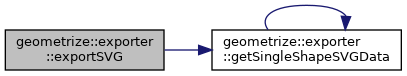std::uint16_t geometrize::exporter::flip16 ( const std::uint16_t & v )
inline
15 {
16  return (v >> 8) | (v << 8);
17 }

Here is the caller graph for this function: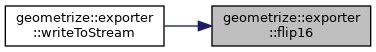std::uint32_t geometrize::exporter::flip32 ( const std::uint32_t & v )
inline
20 {
21  return (v & 0xFF000000) >> 0x18 | (v & 0x000000FF) << 0x18 | (v & 0x00FF0000) >> 0x08 | (v & 0x0000FF00) << 0x08;
22 }

Here is the caller graph for this function: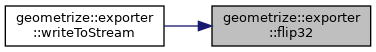std::string geometrize::exporter::getSingleShapeSVGData ( const geometrize::rgba & color, const geometrize::Shape & shape, SVGExportOptions options = `SVGExportOptions{}` )

getSvgShapeData Gets the SVG data for a single shape. This is just the <rect>/<path> etc block for the shape itself, not a complete SVG image.

Parameters
 color The color of the shape. shape The shape to convert to SVG data. options additional options used by the exporter.
Returns
The SVG shape data for the given shape.
241 {
242  return ::getSingleShapeSVGData(color, shape, options);
243 }
std::string getSingleShapeSVGData(const geometrize::rgba &color, const geometrize::Shape &shape, SVGExportOptions options)
getSvgShapeData Gets the SVG data for a single shape. This is just the <rect>/<path> etc block for th...
Definition: svgexporter.cpp:240

Here is the caller graph for this function: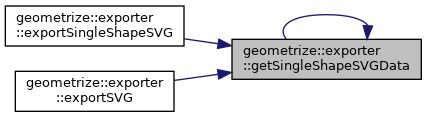void geometrize::exporter::writeToStream ( std::ostringstream & stream, const std::uint8_t & t )
inline
15 {
16  stream.write(reinterpret_cast<const char*>(&t), sizeof(std::uint8_t));
17 }

Here is the caller graph for this function: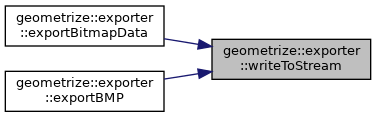void geometrize::exporter::writeToStream ( std::ostringstream & stream, const std::uint32_t & t )
inline
31 {
32  if(bigEndian()) {
33  const std::uint32_t flipped{flip32(t)};
34  stream.write(reinterpret_cast<const char*>(&flipped), sizeof(std::uint32_t));
35  } else {
36  stream.write(reinterpret_cast<const char*>(&t), sizeof(std::uint32_t));
37  }
38 }
std::uint32_t flip32(const std::uint32_t &v)
Definition: bitmapexporter.cpp:19
bool bigEndian()
Definition: bitmapexporter.cpp:24

Here is the call graph for this function: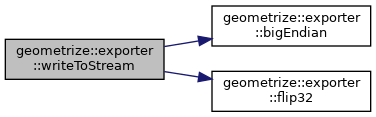void geometrize::exporter::writeToStream ( std::ostringstream & stream, const std::uint16_t & t )
inline
41 {
42  if(bigEndian()) {
43  const std::uint16_t flipped{flip16(t)};
44  stream.write(reinterpret_cast<const char*>(&flipped), sizeof(std::uint16_t));
45  } else {
46  stream.write(reinterpret_cast<const char*>(&t), sizeof(std::uint16_t));
47  }
48 }
std::uint16_t flip16(const std::uint16_t &v)
Definition: bitmapexporter.cpp:14
bool bigEndian()
Definition: bitmapexporter.cpp:24

Here is the call graph for this function: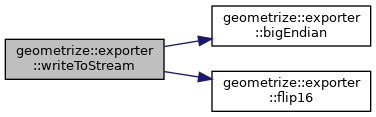## Variable Documentation

 const std::string geometrize::exporter::SVG_STYLE_HOOK = "::svg_style_hook::"
static

SVG_STYLE_HOOK A hook that an SVG exporter should use to augment shape styling produced by the getSvgShapeData method.